Understanding the number system that governs our daily lives may be learned through arithmetic. To count something based on numbers, we need to have some very fundamental understanding. Whether we consider ourselves mathematicians or not, arithmetic is regarded as the basic foundation of mathematics.

It contains a wide variety of formulae and concepts that enable us to do certain computations efficiently. Everyone with an interest in mathematics should thus learn it. The chapter 1 arithmetic sequence answer from the Kerala SSLC Mathematics textbook is available on this website.

Free PDF download available at keralanotes.com for the Kerala SSLC 10th standard Mathematics chapter 1 Syllabus Arithmetic Sequences textbook answer. Here, we've included all of the solutions to the Arithmetic Sequences questions according to the most recent Kerala Math Class 10 curriculum.

 Board SCERT, Kerala Text Book SCERT Based Class SSLC Subject Maths Solution Chapter Chapter 1 Chapter Name Arithmetic Sequences Category Kerala SSLC

## Kerala Syllabus SSLC Class 10 Maths Textbook Solution Chapter 1 Arithmetic Sequences

### Chapter 1  Arithmetic Sequences Textbook Solution

###### Exercise Pg. 10
Question 1.

Look at these triangles with dots. How many dots are there in each?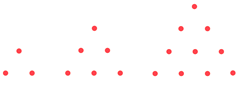Compute the number of dots needed to make the next two triangles.

The number of dots in first 3 triangles are 3, 6 and 10 respectively.

The difference between the first two terms is 6-3 = 3

And 10-6 = 4

The series continues 3, 4, 5…

Hence, this forms an AP with first term as 3 and common difference as 1

Next two terms are 4 + 1 = 5

And 5 + 1 = 6

So 4th pattern has 10 + 5 = 15 dots

5th pattern has 15 + 6 = 21 dots

Question 2.

Make the following number sequences from the sequence of equilateral triangles, squares, regular pentagons and so on, of regular polygons.

Number of sides – 3,4,5…

Sum of interior angles –

Sum of exterior angles –

One interior angle –

One exterior angle –

For the following sequences, let

a = First term of sequence

d = common difference if the sequence is an AP

a) Number of sides – 3,4,5,6,7…. And so on is an Arithmetic Progression.

Where a = 3 and d = 1

b) Sum of interior angles of a regular polygon with n sides = (n-2)×180

Putting n = 3, 4, 5, 6… and so on

The required sequence is 180,360,540,720… and so on.

An AP with a = 180 and d = 180

c) Sum of exterior angles of any closed polygon is 360

Hence the sequence is 360,360,360… and so on i.e. AP with a = 360 and d = 0

d) One Interior angle = (Sum of all interior angles)/(No. of sides)

=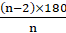Putting n = 3, 4, 5… and so on…

The sequence is 60, 90, 108, 120… and so on. Here, the sequence is not an AP

e) One exterior angle =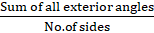(∵ no. of exterior angles = no. of sides)

=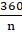Putting n = 3, 4, 5… and so on

The sequence is 120, 90, 72, 60… and so on which is clearly not an AP.

Question 3.

Write down the sequence of natural numbers leaving remainder 1 on division by 3 and the sequence of natural numbers leaving remainder 2 on division by 3.

Dividend = ( Divisor × Quotient) + Remainder

Here, remainder = 1

Divisor = 3

Dividend = ?

On taking quotient as 1, 2, 3 and so on…

Dividend = 4, 7, 10… and so on which is an AP with

a = 4 and d = 3

Now, for 2nd case –

remainder = 2

Divisor = 3

Dividend = ?

On taking quotient as 1,2,3 and so on…

Dividend = 5,8,11… and so on which is an AP with

a = 5 and d = 3

Question 4.

Write down the sequence of natural numbers ending in 1 or 6 and describe it in two other ways.

Sequence of natural numbers ending in 1 or 6 is,

1, 6, 11, 16, 21, 26, 31, 36, …

It can also be described as,

Arithmetic sequence with first term as 1 and common difference as 5

Or

Sequences of natural numbers, which leave 1 as the remainder when divided by 5.

Question 5.

One cubic centimeter of iron weight 7.8 grams. Write as sequences, the volumes of weights of iron cubes of sides 1 centimetre, 2 centimetres and so on.

Volume of cube = side × side × side

For side = 1, Volume = 1 cc.

For side = 2, Volume = 8 cc.

For side = 3, Volume = 27 cc.

Hence, the sequence is 1, 8, 27… and so on which is clearly not an AP

###### Questions Pg-14
Question 1.

Write the algebraic expression for each of the sequences below:

i) Sequence of odd numbers

ii) Sequence of natural numbers which leave remainder 1 on division by 3.

iii) The sequence of natural numbers ending in 1.

iv) The sequence of natural numbers ending in 1 or 6.

i) Sequence of odd numbers-

Odd numbers are those which are not divisible by 2

i.e. 1, 3, 5… and so on

i.e. (2-1),(4-1),(6-1)…and so on

Thus Sn = 2n-1, where n = 1, 2, 3… and so on

ii) Sequence of natural numbers which leave remainder 1 on division by 3. -

Dividend = (Divisor × Quotient) + (Remainder)

Here, Divisor = 3

Remainder = 1

For getting the required sequence, putting quotient as 1, 2, 3… and so on

Dividend = 4,7,10…

i.e. Sn= 3n + 1

iii) The sequence of natural numbers ending in 1.

Natural numbers ending in 1 are 1, 11, 21… and so on

These can be written as (10-9),(20-9),(30-9),… and so on

Hence, the sequence Sn = 10n-9 where n = 1, 2, 3… and so on

iv) The sequence of natural numbers ending in 1 or 6.

The natural numbers ending in 1 or 6 are 1, 6, 11, 16, 21…and so on

Observing the pattern, these numbers can be written as (5-4), (10-4), (15-4),…and so on

Hence, the sequence Sn = 5n-4, where n = 1, 2, 3… and so on

Question 2.

For the sequence of regular polygons starting with an equilateral triangle, write the algebraic expressions for the sequence of the sums of interior angles, the sums of the exterior angles, the measures of an interior angle, and the measures of an exterior angle.

a) Sum of interior angles of any n sided regular polygon = (n-2) × 180 (for n>2)

Hence, Sn = (n-2) × 180

b) Sum of exterior angles of any regular polygon is 360

Hence, Sn= 360 (independent of n)

c) Measure of an interior angle = (Sum of all interior angles) ÷ (No. of sides) (∵ no. of angles = no. of sides)

Sn = ((n-2) × 180) ÷ n

d) Measure of an exterior angle = (Sum of all exterior angles) ÷ (no. of sides)

= 360÷n

Sn = 360/n; where n = 1, 2, 3… and so on

Question 3.

Look at these pictures –The second picture is obtained by removing the small triangle formed by joining the midpoints of the first triangle. The third picture is got by removing such a middle triangle from each of the red triangles of the second picture.

i) How many red triangles are there in each picture?

ii) Taking the area of the first triangles as 1, compute the area of a small triangle in each picture.

iii) What is the total area of all the red triangles in each picture?

iv) Write the algebraic expressions for these three sequences obtained by continuing this

process.

i) How many red triangles are there in each picture?

→ In 1st picture, 1 red triangle

In 2nd picture, 3 red triangles

In 3rd picture, 9 red triangles

S1 = 1, 3, 9…

ii) Taking the area of the first triangles as 1, compute the area of a small triangle in each

picture.

→ In 2nd picture, each triangle is divided into 4 parts in which there are 3 red and 1`white triangle.

Hence, area of each small triangle = (1÷ 4) = 0.25 sq. units

In 3rd picture, each smaller triangle from the 2nd figure, except the middle triangle is further divided into further 4 equal parts.

Hence, area of each small triangle = (0.25/4) = 0.625 sq. units

S2 = 1, 0.25, 0.625…

iii) What is the total area of all the red triangles in each picture?

→ In 1st triangle, only red triangle is present.

In 2nd triangle, 3 red and 1 white triangle is present each of equal areas = 0.25 sq. units

∴ Area of red triangles = 3 × 0.25 = 0.75 sq. units

In 3rd picture, each smaller triangle from the 2nd figure, except the middle triangle is further divided into further 4 equal parts.

Area of all red triangles = 9 × 0.0625 = 0.5625

S3 = 1, 0.75, 0.5625…

iv) Write the algebraic expressions for these three sequences obtained by continuing this process.

For 1st question, the sequence is 1, 3, 9…

Hence, the expression is 3n-1 , where n = 1,2,3…and so on

For 2nd question, the sequence is 1, 0.25, 0.625…

Hence, the expression is (1÷4) (n-1) = (0.25)n-1 ,where n = 1, 2, 3… and so on

For 3rd question, the sequence is 1,0.75,0.675…

Here, the expression is product of above 2 expressions

i.e. 3n-1 × 0.25n-1 = 0.75n-1

###### Questions Pg-17
Question 1.

Check whether each of the sequences given below is an arithmetic sequence. Give reasons.

For the arithmetic sequences, write the common difference also.

i) Sequence of odd numbers

ii) Sequence of even numbers

iii) Sequence of fractions got as half the odd numbers

iv) Sequence of powers of 2

v) Sequence of reciprocals of natural numbers

i) Sequence of odd numbers

→ Odd numbers are those which are not divisible by 2

i.e. 1,3,5,7…

As, can be seen from the above sequence, it is an Arithmetic Progression.

Common difference = 3-1 = 5-3 = 2

ii) Sequence of even numbers

→ Even numbers are those which are divisible by 2

I.e. 2,4,6…

As, can be seen from the above sequence, it is an Arithmetic Progression.

Common difference = 4-2 = 6-4 = 2

iii) Sequence of fractions got as half the odd numbers

→ Odd numbers are those which are not divisible by 2

i.e. 1,3,5,7…

The sequence mentioned is half the odd numbers…

I.e. 1/2, 3/2, 5/2, 7/2,…

As, can be seen from the above sequence, it is an Arithmetic Progression.

Common difference =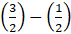=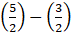= 1

iv) Sequence of powers of 2

→ The sequence of powers of 2 is as follows

21,22,23,… and so on

i.e. 2,4,8,…

Difference of second and first term = 4-2 =2

Difference of third and second term = 8-4 =4

As the difference is not same, it is not an AP.

v) Sequence of reciprocals of natural numbers

→ Natural numbers are those starting from 1 with common difference as 1

Sequence of reciprocals of natural numbers is as follows –

1/1, 1/2, 1/3 , 1/4 , 1/5… and so on

Difference of second and first term = (1/2)-(1/1) = -1/2

Difference of third and second term = (1/3) – (1/2) = -1/6

As the difference is not same, it is not an AP.

Question 2.

Look at these pictures.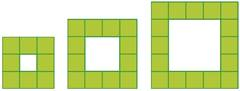If the pattern is continued, do the numbers of coloured squares from an arithmetic sequence? Give reasons.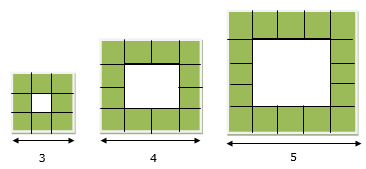No. of coloured squares in

Figure 1 – 8

Figure 2 – 12

Figure 3 – 16

As can be seen from the figures, the side of each coloured square increases linearly from 3,4,5… and so on. Hence, it is an AP

With common difference = 12-8 = 16-12 = 4

Question 3.

See this pictures below –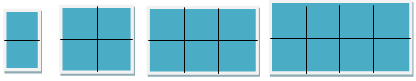i) How many small squares are there in each rectangle?

ii) How many large squares?

iii) How many squares in all?

Continuing this pattern, is each such sequence of numbers, an arithmetic sequence?

i) How many small squares are there in each rectangle?

→ No. of small squares in

Rectangle 1 - 2

Rectangle 2 - 4

Rectangle 3 - 6

Rectangle 4 - 8

As is evident, it forms an AP with common difference = 4-2 =2

ii) How many large squares?

→ No. of large squares in

Rectangle 1 - 0

Rectangle 2 - 1 (intersection of all 4 small squares)

Rectangle 3 - 2 (2 same size overlapping squares as in rectangle 2)

Rectangle 4 - 3

As is evident, it forms an AP with common difference = 1-0 =1

iii) How many squares in all?

→ All squares = small squares + large squares

No. of all squares in

Rectangle 1 - 2 + 0 = 2

Rectangle 2 - 4 + 1 = 5

Rectangle 3 - 6 + 2 = 8

Rectangle 4 - 8 + 3 = 11

As is evident, it forms an AP with common difference = 2+1 = 3

Question 4.

In this picture, the perpendiculars to the bottom line are equally spaced. Prove that, continuing like this, the lengths of perpendiculars form an arithmetic sequence.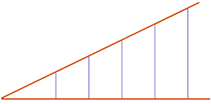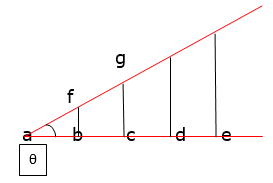In Δ fab , Δ gac , and so on…

The subtended angle θ is same

∴ tanθ = constant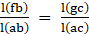Now l(ab)=l(bc)=l(cd)… given

∴ l(ac)=2× l(ab)

For, tanθ = constant

l(gc)=2× l(fb)

l(hd)=3× l(fb)

Hence, lengths fb,gc,hd…are in AP

i.e. the length of perpendiculars are in AP.

Question 5.

The algebraic expression of a sequence is.

Xn = n3 – 6n2+ 13n – 7

Is it an arithmetic sequence?

For it to be an AP, xn+1 – xn = constant

xn+1-xn = {(n+1)3-6(n+1)2+13(n+1)-7}-{ n3 – 6n2+ 13n – 7}

= (n+1)3-n3-6{(n+1)2-n2}+13(n+1-n)-7+7

= (n+1-n)(n2+2n+1+n2+n+n2)-6(2n+1)+13

= 1× (3n2+3n+1)-12n+7

= 3n2-9n+8

Which is not independent of n. Hence, the sequence is not an AP.

###### Questions Pg-21
Question 1.

In each of the arithmetic sequences below, some terms are missing and their positions are marked with ∘. Find them.

i) 24, 42, ∘, ∘, … ii) ∘, 24, 42, ∘, …

iii) ∘, ∘, 24, 42, … iv) 24, ∘, 42, ∘, …

v) ∘, 24, ∘, 42, … vi) 24, ∘, ∘, 42, …

Let a = first term of arithmetic sequence

a2 , a3 , a4… are second, third, fourth terms and so on

d = common difference

i) Here, a = 24

a2 = 42

d = 42-24 = 18

a3 = 42 + 18 = 60

a4 = 60 + 18 = 78

ii) Here, a2 = 24

a3 = 42

d = 42-24 = 18

a = a2 – d = 24-18 = 6

a4 = 42 + 18 = 60

iii) Here, a3 = 24

a4 = 42

d = a4 – a3 = 42-24 = 18

a2 = 24 – 18 = 6

a = 6-18 = -12

iv) Here, a = 24

a3 = 42

a3 = a + 2d

Solving, d = 9

∴ a2 = 24 + 9 = 33

a4 = 42 + 9 = 51

v) Here, a2 = 24

a4 = 42

a4 = a + 3d …(eq)1

and,a2 = a + d …(eq)2

Eliminating a;

d = 9

a = 24-9 = 15

a3 = 24 + 9 = 33

vi) Here, a = 24

a4 = 42

a4 = a + 3d

Solving, d = 6

a2 = 24 + 6 = 30

a3 = 30 + 6 = 36

Hence, all the answers are found.

Question 2.

The terms in two positions of some arithmetic sequences are given below. Write the first five terms of each:

i) 3rd term 34

6th term 67

ii) 3rd term 43

6th term 76

iii) 3rd term 2

5th term 3

iv) 4th term 2

7th term 3

v) 2nd term 5

5th term 2

i) a3 = 34

a6 = 67

a3 = a + 2d and a6 = a + 5d

Solving,a6 = a3 + 3d

∴ d = 11

a2 = 34-11 = 23

a = 23-11 = 12

a4 = 34 + 11 = 45

a5 = 45 + 11 = 56

First 5 terms are 12,23,34,45 and 56

ii) a3 = 43

a6 = 76

a3 = a + 2d and a6 = a + 5d

Solving,a6 = a3 + 3d

∴ d = 11

a2 = 43-11 = 32

a = 32-11 = 21

a4 = 43 + 11 = 54

a5 = 54 + 11 = 65

First 5 terms are 21,32,43,54 and 65

iii) a3 = 2

a5 = 3

a3 = a + 2d and a5 = a + 4d

Solving, a5 = a3 + 2d

d = 0.5

a2 = 2-0.5 = 1.5

a = 1.5-0.5 = 1

a4 = 2 + 0.5 = 2.5

First 5 terms are 1,1.5,2,2.5 and 3

iv) a4 = 2

a7 = 3

a4 = a + 3d

a7 = a + 6d

Solving,a7 = a4 + 3d

d =a3 =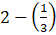=a2 =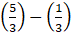=a =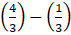= 1

a5 = 2 +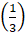=First 5 terms are 1,4/3,5/3,2 and 7/3

v) a2 = 5

a5 = 2

a2 = a + d

a5 = a + 4d

Solving,a5 = a2 + 3d

d = -1

a = 5-(-1) = 6

a3 = 5-1 = 4

a4 = 4-1 = 3

a5 = 3-1 = 2

First 5 terms are 6,5,4,3,2.

Hence solved.

Question 3.

The 5th term of an arithmetic sequence is 38 and the 9th term is 66. What is its 25th term?

Given:

a5 = 38 and,

a9 = 66

We know, nth term is given as,

[an = a + (n-1)× d]

⇒ a5 = a + 4d ..(1)

And, a9 = a + 8d …(2)

Solving eq(1) and eq(2), we get,

a9 = a5 + 4d

⇒ 66 = 38 + 4d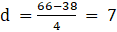.

Now, from eq (1),

a5 = a + 4d

⇒ 38 = a + 4(7)

⇒ a = (38-(4×7))

⇒ a = 38 – 28

⇒ a = 10

And,

25th term, a25 = a + 24d

= 10 + (24×7)

= 178

Hence, 25th term is 178

Question 4.

Is 101 a term of the arithmetic sequence 13, 24,35,…? What about 1001?

a = 13

a2 = 24

a3 = 35

d = 35-24 = 11

Case i)

an = 101

an = 13 + (n-1)d

Solving, n = 9

∴ 101 is the term of this AP.

Case ii)

an = 1001

an = 13 + (n-1)d

Solving, n = 90.8

∴ 1001 is not the term of this AP.

Question 5.

How many three-digit numbers are there, which leave a remainder 3 on division by 7?

Dividend = (divisor× quotient) + remainder

Here, divisor = 7

Remainder = 3

First 3 digit no. is 100

For, dividend = 100, quotient = 13.6…

Hence, first 3 digit no. divisible by 7 with remainder 3 comes with quotient>13.6… = 14

When quotient = 14, dividend = 101

Last 3 digit no. divisible by 7 with remainder 3 = 997

an = 997

a = 101

d = 7

an = a + (n-1)× d

∴ n-1 = 128

∴ n = 129

Hence,129 numbers are present.

Question 6.

Fill up the empty cells of the square below such that the numbers in each row and column from arithmetic sequences:

What if we use other numbers instead of 1, 4 28 and 7?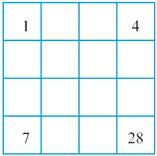For 1st row,

a = 1 a4 = 4

a4 = a + 3d

∴ d = 1

a2 = 1 + 1 = 2

A12 = 2

a3 = 2 + 1 = 3

A13 = 3

For 1st column,

a = 1 a4 = 7

a4 = a + 3d

∴ d = 2

a2 = 1 + 2 = 3

A21 = 3

a3 = 3 + 2 = 5

A31 = 5

For 4th row,

a = 7 a4 = 28

a4 = a + 3d

∴ d = 7

a2 = 7 + 7 = 14

A42 = 14

a3 = 14 + 7 = 21

A43 = 21

For 4th column,

a = 4 a4 = 28

a4 = a + 3d

∴ d = 8

a2 = 4 + 8 = 12 ∴ A24 = 12

a3 = 12 + 8 = 20 ∴ A34 = 20

For 2nd row,

a = 3 a4 = 12

a4 = a + 3d

∴ d = 3

a2 = 3 + 3 = 6 ∴ A42 = 6

a3 = 6 + 3 = 9 ∴A23 = 9

For 3rd row,

a = 5 a4 = 20

a4 = a + 3d

∴ d = 5

a2 = 5 + 5 = 10

A32 = 10

a3 = 10 + 5 = 15

A33 = 15

If we use numbers other than 1,4,7,and 28 it is not guaranteed that the grid will be completely filled such that each row and column is in AP.

Question 7.

In the table below, some arithmetic sequences are given with two numbers against each. Check whether each belongs to the sequence or not.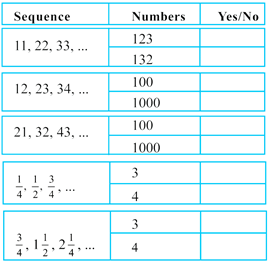a) a = 11

d = 22-11 = 11

i) let an = 123

an = a + (n-1)×d

∴ n = 11.18

Since, n is not an integer, 123 is not a part of sequence

ii) let an = 132

an = a + (n-1)×d

∴ n = 12

Since, n is an integer, 132 is a part of sequence

b) a = 12

d = 23-12 = 11

i) let an = 100

an = a + (n-1)×d

∴ n = 9

Since, n is an integer, 100 is a part of sequence

ii) let an = 1000

an = a + (n-1)×d

∴ n = 90.81

Since, n is not an integer, 1000 is not a part of sequence

c) a = 21

d = 32-21 = 11

i) let an = 100

an = a + (n-1)×d

∴ n = 8.18

Since, n is not an integer, 100 is not a part of sequence

ii) let an = 1000

an = a + (n-1)×d

∴ n = 90

Since, n is an integer, 1000 is a part of sequence

d) a = 1/4

d = (1/2)-(1/4) = 1/4

i) let an = 3

an = a + (n-1)×d

∴ n = 12

Since, n is an integer, 3 is a part of sequence

ii) let an = 4

an = a + (n-1)×d

∴ n = 16

Since, n is an integer, 4 is a part of sequence

e) a = 3/4

d = (3/2)-(3/4) = 3/4

i) let an = 3

an = a + (n-1)×d

∴ n = 4

Since, n is an integer, 3 is a part of sequence

ii) let an = 4

an = a + (n-1)×d

∴ n = 5.33

Since, n is not an integer, 4 is not a part of sequence

###### Questions Pg-26
Question 1.

Write three arithmetic sequences with 30 as the sum of the first five terms.

Sn = 30 where n = 5

For an AP with a = first term

d = common difference

Sn =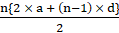Substituting in above equation…

∴ 2a + (5-1) × d = 12

∴ 2a + 4d = 12

∴ a + 2d = 6

i) Let a = 2, hence d = 2

Sequence is 2,4,6,8,10…

ii) Let a = 4, hence d = 1

Sequence is 4,5,6,7,8…

iii) Let a = 6, hence d = 0

Sequence is 6,6,6,6,6…

Hence, 3 sequences are found.

Question 2.

The first term of an arithmetic sequence is 1 and the sum of the first four terms is 100. Find the first four terms.

a = 1 S4 = 100 n = 4

Sn =Substituting everything in above equation,

2a + (4-1) × d = 50

∴ 3d = 48

∴ d = 16

First four terms are 1,17,33,49.

Hence, the answer is written above.

Question 3.

Prove that for any four consecutive terms of an arithmetic sequence, the sum of the two terms on the two ends and the sum of the two terms in the middle are the same.

Let a,b,c and e are four consecutive terms of an AP with d = common difference.

b = a + d

c = a + 2d

e = a + 3d

First term + Last term = a + e = a + (a + 3d) = 2a + 3d …(1)

Second term + Third term = b + c = (a + d) + (a + 2d) = 2a + 3d …(2)

Hence,

First term + Last term = Second term + Third term

Question 4.

Write four arithmetic sequences with 100 as the sum of the first four terms.

Sn = 100 where n = 4

For an AP with a = first term

d = common difference

Sn =∴ 2a + (4-1) × d = 50

∴ 2a + 3d = 50

Let a = 1, hence d = 16

Sequence is 1,17,33,49…

Let a = 4, hence d = 14

Sequence is 4,18,32,46…

Let a = 7 hence d = 12

Sequence is 7,19,31,43…

Question 5.

The 8th term of an arithmetic sequence is 12 and its 12th term is 8. What is the algebraic expression for this sequence?

a8 = 12,

a12 = 8

an = a + (n-1) × d

a8 = a + 7d = 12 …(eq)1

a12 = a + 11d = 8 …(eq)2

(eq)2-(eq)1

4d = -4

d = -1

a = 19

So, sequence is 19,18,17,16…

Question 6.

The Bird problem in Class 8 (The lesson, Equations) can be slightly changed as follows.

One bird said:

“We and we again, together with half of us and half of that, and one more is a natural number”

Write the possible number of birds in order. For each of these, write the sum told by the bird also.

Find the algebraic expression for these two sequences.

Let the possible no of birds be x,

Therefore, according to given condition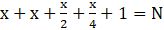Where N is any natural number.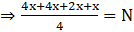⇒ 11x = 4N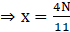Also, no of birds should also be a natural number, therefore N should be a multiple of 11, i.e. N = 11n for any natural no 'n'

Hence possible values of N = 11, 22, 33…

And possible values of x = 4, 8, 12, …

Algebraic expression for N is 11n

And

For x is 4n.

Question 7.

Prove that the arithmetic sequence with first term 1/3 and common difference 1/6 contains all natural numbers.

For the sequence,

a =d =an = a + (n-1) × d

Let p be any natural number

p =+ (n-1) ×=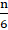+=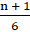6p = n + 1

Thus, for every natural number p, a value of n exists.

Hence, this arithmetic sequence contains all natural numbers.

Question 8.

Prove that the arithmetic sequence with first term 1/3 and common difference 2/3 contains all odd numbers, but no even number.

For the sequence,

a =d =an = a + (n-1) × d

=+ (n-1) ×=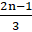= k(2n-1) (where k = constant)

As an is a multiple of (2n-1) and not (2n) it contains all odd numbers but no even number..

Question 9.

Prove that the squares of all the terms of the arithmetic sequence 4, 7, 10, … belong to the sequence.

a = first term = 4

d = common difference = 7-4 = 3

an = a + (n-1) × d

an = 4 + 3n-3 = 3n + 1

(an)2 = (3n + 1)2 = 9n2 + 6n + 1 = 3n(3n + 2) + 1 = 3{n(3n + 2)} + 1

As, (an)2 is also of the form 3n + 1, the squares of all the terms of the arithmetic sequence 4, 7, 10, … belong to the sequence.

Question 10.

Prove that the arithmetic sequence 5, 8, 11, … contains no perfect squares.

a = 5

d = 8-5 = 3

an = a + (n-1) × d

an = 5 + (n-1) × 3

∴ an = 3n + 2

Let p be a natural number

p2 = 3n + 2

n =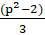Now, for all integers from 0 to 9(i.e. p from 0 to 9), n does not come out to be an integer.

Hence, the arithmetic sequence 5, 8, 11, … contains no perfect squares.

Question 11.

The angles of a pentagon are in arithmetic sequence. Prove that its smallest angle is greater than 36°.

Sum of all angles of a regular polygon with n sides = (n-2) × 180

Let a,a + d,a + 2d,a + 3d,a + 4d are 5 terms of an AP.

Sum of all angles = 5a + 10d = (5-2) × 180 = 540

∴ a + 2d = 108

The minimum angle will be obtained when a = d.

a + 2a = 3a = 108

∴ a = 36 is the minimum angle.

Question 12.

Write the whole numbers in the arithmetic sequence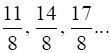. Do they form an arithmetic sequence?

Let a =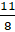a2 =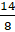a3 =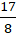a2-a =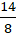-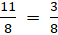a3-a2 =-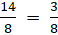As a3-a2 = a2-a

Hence, the common difference is same, i.e. the sequence forms an AP.

###### Questions Pg-32
Question 1.

Find the sum of the first 25 terms of each of the arithmetic sequences below.

11, 22, 33, …

a = 11 = first term

d = 22-11 = 11 = common difference

Sn =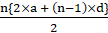Here, n = 25

∴ S25 =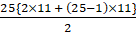= 25× 11× 13

= 3575

Question 2.

Find the sum of the first 25 terms of each of the arithmetic sequences below.

12, 23, 34, ….

a = 12

d = 23-12 = 11

Sn =Here, n = 25

∴ S25 =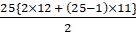= 25× 24× 6

= 3600

Question 3.

Find the sum of the first 25 terms of each of the arithmetic sequences below.

21, 32, 43, …

a = 21

d = 32-21 = 11

Sn =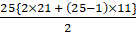Here, n = 25

∴ S25 = 3825

Question 4.

Find the sum of the first 25 terms of each of the arithmetic sequences below.

19, 28, 37, …

a = 19

d = 28-19 = 9

Sn =Here, n = 25

∴ S25 =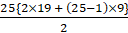= 3175

Question 5.

Find the sum of the first 25 terms of each of the arithmetic sequences below.

1, 6, 11, …

a = 1

d = 6-1 = 5

Sn =Here, n = 25

∴ S25 =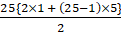= 1525

Hence, the sum of first 5 terms for each sequence is found out.

Question 6.

What is the difference between the sum of the first 20 terms and the next 20 terms of the arithmetic sequence 6, 10, 14, …?

For the arithmetic sequence,

a = 6

d = 10-6 = 4

Sn =…(eq)1

Sum of first 20 terms = S20

Sum of next 20 terms = S40-s20

Substituting values in eq1

S20 =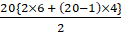= 10× 88

= 880

S40 =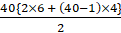= 20× 168

= 3360

Sum of first 20 terms = S20 = 880

Sum of next 20 terms = S40-s20 = 3360-880 = 2480

∴ Difference between sum of first 20 terms = 2480-880 = 1600

And next 20 terms

Question 7.

Calculate the difference between the sum of the first 20 terms of the arithmetic sequences 6, 10, 14, … and 15, 19, 23, …

Case i)

a = 6

d = 10-6 = 4

n = 20

Sn1 == 10×88

= 880

Case ii)

a = 15

d = 19-15 = 4

n = 20

Sn2 =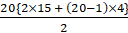= 10×106

= 1060

The difference between the sum of the first 20 terms of the arithmetic sequences 6, 10, 14, … and 15, 19, 23, … = Sn1 – Sn2

= 1060- 880

= 180

Question 8.

Find the sum of all three digit numbers, which are multiples of 9.

Three digit numbers which are multiples of 9 are in AP

The sequence is 108,117..999

a = 108

an = 999

d = 9

an = a + (n-1)×d

999 = 108 + (n-1)× 9

∴ n = 100

Sn =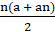=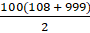= 50× 1107

= 55350

Question 9.

Find n is the equation 52 × 54 × 56 × … × 52n = (0.008)-30.

am× an = am + n

LHS = 52 × 54 × 56 × … × 52n

= 52 + 4 + 6 + …2n

= 52(1 + 2 + 3 + …n)

{1 + 2 + 3 + …n =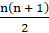}

=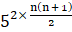= 5n(n + 1)

RHS = (0.008)-30 = (8× 10-3)-30

= (23× (2×5)-3)-30

= (23× 2-3× 5-3)-30

= 590

∵ (am)n = amn

LHS = RHS

∴ 5n(n + 1) = 590

∴ n(n + 1) = 90

∴n2 + n-90 = 0

Solving for n,

n = 9 and n = -10

But, n<0 is not possible

Hence, n = 9

Question 10.

The expressions for the sum to n terms of some arithmetic sequences are given below. Find the expression for the nth term of each:

n2 + 2n

Sn = n2 + 2n

an + 1 = Sn + 1 - Sn

= {(n + 1)2 + 2(n + 1)}-{n2 + 2n}

= n2 + 4n + 4-n2-2n

= 2n + 4

= 2(n + 1) + 2

∴ an = 2n + 2

Question 11.

The expressions for the sum to n terms of some arithmetic sequences are given below. Find the expression for the nth term of each:

2n2 + n

Sn = 2n2 + n

an + 1 = Sn + 1 - Sn

= {2(n + 1)2 + (n + 1)}- {2n2 + n}

= (2n2 + 4n + 4 + n + 1)-(2n2 + n)

= 4n + 5

= 4(n + 1) + 1

∴ an = 4n + 1

Question 12.

The expressions for the sum to n terms of some arithmetic sequences are given below. Find the expression for the nth term of each:

n2 – 2n

Sn = n2 – 2n

an + 1 = Sn + 1-Sn

= {(n + 1)2-2(n + 1)}- (n2 – 2n)

= n2 + 2n + 1-2n-2-n2 + 2n

= 2n-1

= 2(n + 1)-3

an = 2n-3

Question 13.

The expressions for the sum to n terms of some arithmetic sequences are given below. Find the expression for the nth term of each:

2n2 – n

Sn = 2n2 - n

an + 1 = Sn + 1 - Sn

= {2(n + 1)2-(n + 1)}- {2n2 - n}

= (2n2 + 4n + 2-n-1)-(2n2-n)

= 4n + 1

= 4(n + 1)-3

an = 4n-3

Question 14.

The expressions for the sum to n terms of some arithmetic sequences are given below. Find the expression for the nth term of each:

n2 – n

Sn = n2-n

an + 1 = Sn + 1-Sn

= {(n + 1)2-(n + 1)}-(n2–n)

= n2 + 2n + 1-n-1-n2 + n

= 2n

= 2(n + 1)-2

an = 2n-2

Question 15.

Calculate in head, the sums of the following arithmetic sequences.

i) 51 + 52 + 53 + … + 70

ii)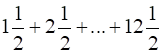iii)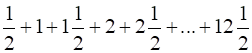(i) Here, we will use formula in which we can calculate sum of AP by first term(a) and last term(an) i.e.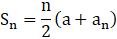Where n is no of terms

Clearly first term, a = 51

Last term, an = 70

And no of terms, n = 70 - 51 + 1 = 20

Hence, sum is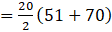= 10(121)

= 1210

(ii)

Here, we will use formula in which we can calculate sum of AP by first term(a) and last term(an) i.e.Where n is no of terms

Clearly first term,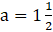Last term,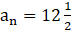Common difference,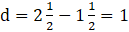Therefore, we can calculate no of terms usinh,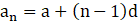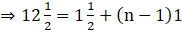⇒ 11 = n - 1

⇒ n = 12

Hence, we have sum as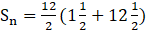⇒ Sn = 6(14) = 84

(iii)

Here, we will use formula in which we can calculate sum of AP by first term(a) and last term(an) i.e.Where n is no of terms

Clearly first term,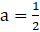Last term,Common difference,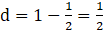Therefore, we can calculate no of terms usinh,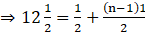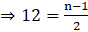⇒ n = 25

Hence, we have sum as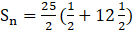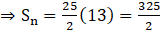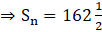Question 16.

The sum of the first 10 terms of an arithmetic sequence is 350 and the sum of the first 5 terms is 100. Write the algebraic expression for the sequence.

S10 = 350

S5 = 100

Sn =When n = 10,

S10 = 350 =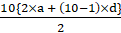∴ 2a + 9d = 70 …(eq)1

S5 = 100 =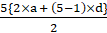∴ a + 2d = 20 …(eq)2

Solving equations 1 and 2…

d = 6

a = 8

Hence, the sequence is 8,14,20…

an = a + (n-1)×d = 8 + (n-1)×6 = 6n + 2

Question 17.

Prove that the sum of any number of terms of the arithmetic sequence 16, 24, 32, … starting from the first, added to 9 gives a perfect square.

a = 16

d = 24-16 = 8

Let number of terms = n

Sn ==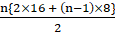= n(12 + 4n)

Sn + 9 = 4n2 + 12n + 9 = (2n + 3)2

Hence, the sum of any number of terms of the arithmetic sequence 16, 24, 32, …. starting from the first, added to 9 gives a perfect square.

Question 18.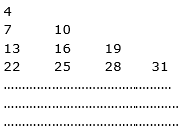Write the next two lines of the pattern above. Calculate the first and last terms of the 20th line.

The first terms of each line are 4,7,13,22…

The difference between each consecutive term is

7-4 = 3

13-7 = 6

22-13 = 9

i.e. 3,6,9… in AP

For 4th line,

First term = 22 + 12 = 34 , d = 3

For 5th line

First term = 34 + 15 = 49 , d = 3

Also from observation, nth line has n terms

Hence, 4th line

34,37,40,43

And 5th line

49,52,55,58,61

The sequence of common difference is

The difference between the first terms of each line forms an AP which is

3,6,9…

For first term of 20th line, sum of 19 terms of above sequence to be added to first term of first line.

Sum of 19 terms =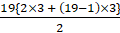= 570

For 20th line,

First term = 4 + 570 = 574

It has 20 terms

Last term = 574 + 19×3 = 574 + 57 = 631

### SSLC Maths Textbook Solution

Feel free to comment and share this article if you found it useful. Give your valuable suggestions in the comment session or contact us for any details regarding the HSE Kerala SSLC class 10 syllabus, Previous year question papers, and other study materials.

We hope the given HSE Kerala Board Syllabus SSLC class 10 Textbook Solutions Chapter Wise Pdf Free Download in both English Medium and Malayalam Medium will help you.

If you have any query regarding the Higher Secondary Kerala SSLC class 10 SCERT syllabus, drop a comment below and we will get back to you at the earliest.

Keralanotes.com      Keralanotes.com      Keralanotes.com      Keralanotes.com      Keralanotes.com